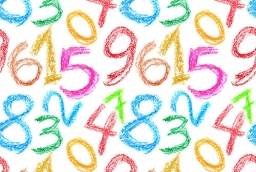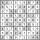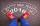# Two numbers 7

The sum of two consecutive even numbers is 30. Find the numbers.

a =  14
b =  16

### Step-by-step explanation:

a+b=30
b = a+2

a+b = 30
a-b = -2

a = 14
b = 16

Our linear equations calculator calculates it.Did you find an error or inaccuracy? Feel free to write us. Thank you!Tips to related online calculators
Do you have a linear equation or system of equations and looking for its solution? Or do you have a quadratic equation?

## Related math problems and questions:

• The sum 16The sum of A and B is 36. The difference between A & B is 8. find the larger number of A.
• Unknown numbersThe sum of two consecutive natural numbers and their triple is 92. Find these numbers.
• Even / odd numbersa / Using variable n write two consecutive odd numbers. b / the sum of three consecutive odd numbers is 333. What are this numbers?
• Nice two numbersWhat two numbers we give a sum of 30 difference of 24 products of 81 and a quotient of 9?
• Three intsThe sum of three consecutive integers is 2016. What numbers are they?
• The sum 8The sum of two numbers is 21. If three times the smaller numbers is two less than twice the larger number, find the two numbers.
• Sum and ratioThe sum and ratio of two numbers is equal to 10. Which numbers are they?
• The sumThe sum of the squares of two immediately following natural numbers is 1201. Find these numbers.
• Odd numbersThe sum of four consecutive odd numbers is 1048. Find those numbers ...
• Ratio of two unknown numbersTwo numbers are given. Their sum is 30. We calculate one-sixth of a larger number and add to both numbers. So we get new numbers whose ratio is 5:7. Which two numbers were given?
• Two numbersThe sum of two numbers is 1. Identify this two numbers if you know that the half of first is equal to the third of second number.
• AlgebrogramSolve algebrogram for sum of three numbers: BEK KEMR SOMR ________ HERCI
• A number 4A number is made up of two digits. The sum of the digits is 11. if the digits are interchanged, the original number is increase by 9. Find the original number
• Sum of sevenThe sum of seven consecutive odd natural numbers is 119. Determine the smallest of them.
• Find twoFind two consecutive natural numbers whose product is 1 larger than their sum. Searched numbers expressed by a fraction whose numerator is the difference between these numbers and the denominator is their sum.
• The largerThe larger of two numbers is nine more than four times the smaller number. The sum of the two numbers is fifty-nine. Find the two numbers.
• AM of three numbersThe number 2010 can be written as the sum of 3 consecutive natural numbers. Determine the arithmetic mean of these numbers.